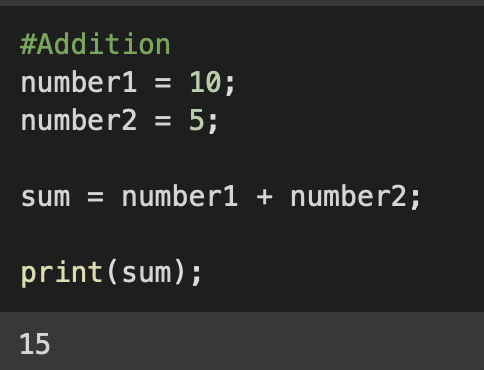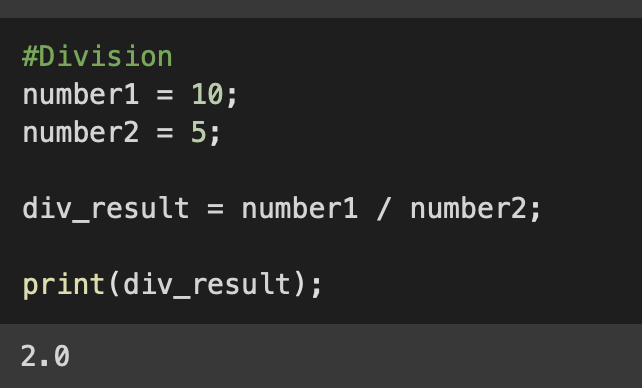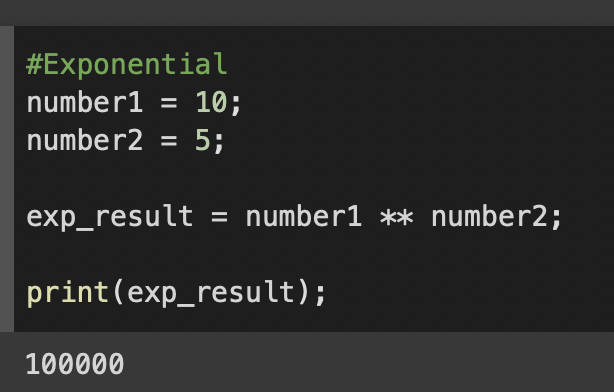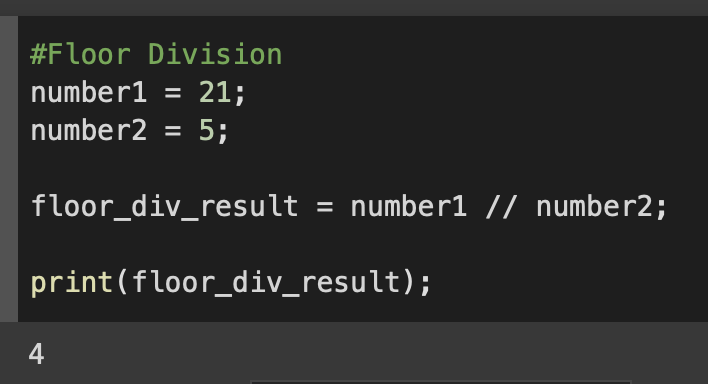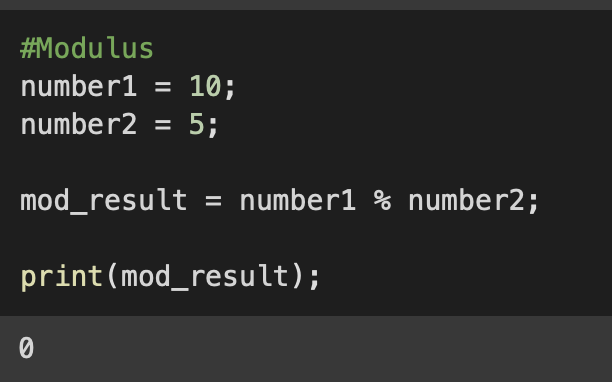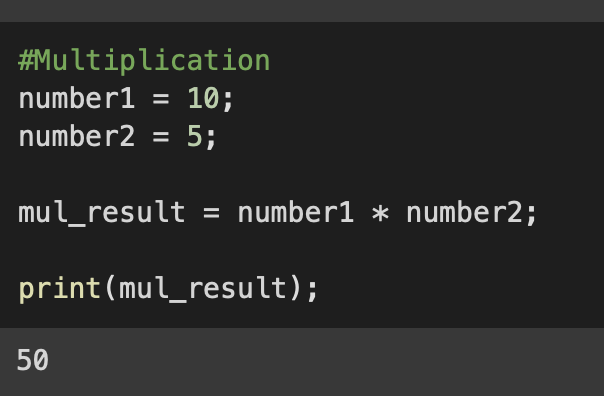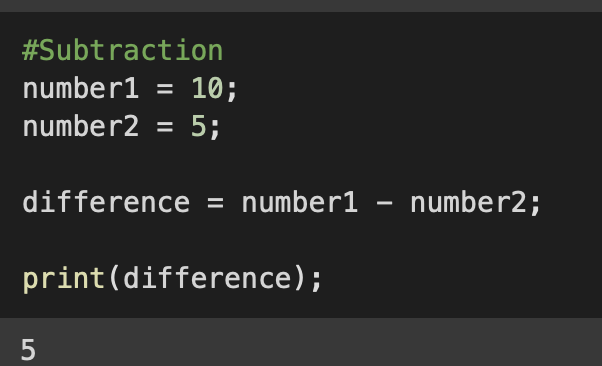# 7 Python Arithmetic Operators with Examples [Tutorial]

## List of Arithmetic Operations in Python:

2. Subtraction: -
3. Multiplication: *
4. Division: /
5. Floor Division: //
6. Modulus: %
7. Exponential: **

## Python Arithmetic Operators Examples

Example:
``````# Python: Arithmetic Operator: Addition (+) Example

number1 = 10;
number2 = 20;

sum = number1 + number2;

print(sum);``````
Output:

30

2. ### Subtraction: -

Example:
``````# Python: Arithmetic Operator: Subtraction (-) Example

number1 = 30;
number2 = 20;

#Subtraction
difference = number1 - number2;

print(difference);``````
Output:

10

3. ### Multiplication: *

Example:
``````# Python: Arithmetic Operator: Multiplication (*) Example

number1 = 10;
number2 = 20;

#Multiplication
mutiplicationResult = number1 * number2;

print(mutiplicationResult);``````
Output:

200

4. ### Division: /

Example:
``````# Python: Arithmetic Operator: Division (/) Example

number1 = 20;
number2 = 10;

#Division
divisionResult = number1 / number2;

print(divisionResult);``````
Output:

10

5. ### Floor Division: //

``````# Python: Arithmetic Operator: Floor Division (//) Example

number1 = 21;
number2 = 4;

#Floor Division
#Using Floor Division you get the rounded nearest whole number as the result.
floorDivisionResult = number1 / number2;

print(floorDivisionResult);``````
Output:

5

6. ### Modulus: %

``````# Python: Arithmetic Operator: Modulus (%) Example

number1 = 10;
number2 = 2;

#Modulus
modulusResult = number1 % number2;

print(modulusResult);``````
Output:

0

7. ### Exponentiation: **

``````# Python: Arithmetic Operator: Exponentiation (**) Example

number1 = 10;
number2 = 5;

#Modulus
exponentialResult = number1 ** number2;

print(exponentialResult);``````
Output:

100000